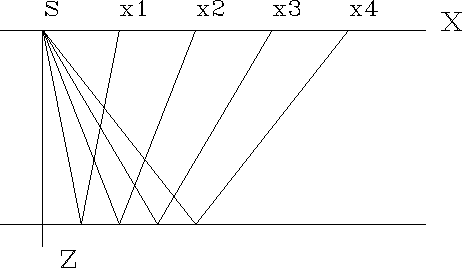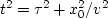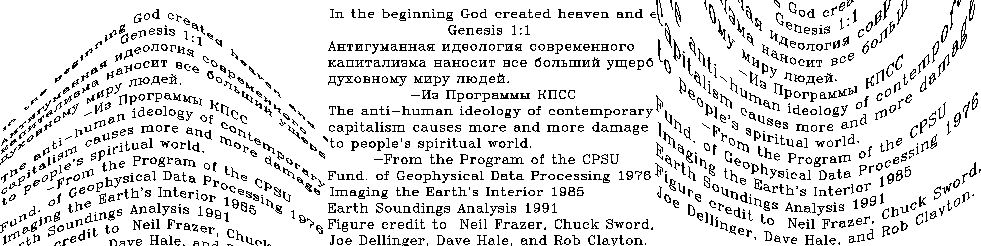Next: Stack Up: NORMAL MOVEOUT AND OTHER Previous: Formal inversion

## Nearest-neighbor NMO

Normal-moveout correction (NMO) is a geometrical correction of reflection data that stretches the time axis so that data recorded at nonzero separation x0 of shot and receiver, after stretching, appears to be at x0=0. See Figure 3.

 geometry Figure 3 A sound emitter at location s on the earth's surface z=0, and rays from a horizontal reflector at depth z reflecting back to surface locations xi.NMO correction is roughly like time-to-depth conversion with the equation v2 t2 = z2 + x02. After the data at x0 is stretched from t to z, it should look like stretched data from any other x (assuming plane horizontal reflectors, etc.). In practice, z is not used; rather, traveltime depthis used, where;so.

To show how surfaces deform under moveout correction, I took a square of text and deformed it according to the NMO correction equation and its inverse. This is shown in Figure 4.frazer
Figure 4
Roughly, NMO takes each panel to the one on its right.

The figure assumes a velocity of unity, so the asymptotes of the hyperbolas lie at.The main thing to notice is that NMO stretches information at wide offsets and early time, whereas modeling, its inverse, squeezes it. More precisely, starting from the center panel, adjoint NMO created the left panel, and NMO created the right panel. Notice that adjoint NMO throws away data at late time, whereas NMO itself throws away data at early time. Otherwise, adjoint NMO in this example is the same as inverse NMO. 1Next: Stack Up: NORMAL MOVEOUT AND OTHER Previous: Formal inversion
Stanford Exploration Project
10/21/1998